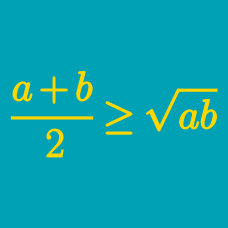Algebra

# QM-AM-GM-HM

Given positive $a_1, a_2, \ldots, a_j,$ let $f$ be defined as

$f(n) = \left(\frac{a_1^n + a_2^n + \cdots + a_j^n}{j}\right)^{1/n}.$

For $m > n$, which of the following is necessarily true?

If the arithmetic mean and quadratic mean (root mean square) of a set of positive numbers are equal, which of the following means must also equal that same value?

What is the smallest $n$ for which

$\left(a^3 + b^3\right)^2 \leq n (a^6 + b^6)$

holds for all positive $a$ and $b$?

What is the largest $n$ for which

$(x + y) (y + z) (x + y + z) \geq \frac{n x^2 y^3 z^2}{(x + y)(y + z)(xy + xz + yz)}$

holds for all positive $x, y, z$?

Suppose the harmonic mean of $5$ positive numbers is equal to $2.$ What is the minimum possible sum of the numbers?

×